Updating search results...

# 7 Results

View
Selected filters:
• WI.Math.Content.HSA-APR.A.1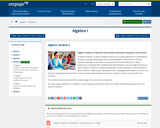Conditional Remix & Share Permitted
CC BY-NC-SA
Rating
0.0 stars

In earlier modules, students analyze the process of solving equations and developing fluency in writing, interpreting, and translating between various forms of linear equations (Module 1) and linear and exponential functions (Module 3). These experiences combined with modeling with data (Module 2), set the stage for Module 4. Here students continue to interpret expressions, create equations, rewrite equations and functions in different but equivalent forms, and graph and interpret functions, but this time using polynomial functions, and more specifically quadratic functions, as well as square root and cube root functions.

Subject:
Algebra
Mathematics
Material Type:
Module
Provider:
New York State Education Department
Provider Set:
EngageNY
09/17/2013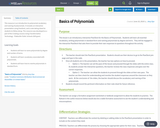Conditional Remix & Share Permitted
CC BY-NC-SA
Rating
0.0 stars

This resource is an introduction to polynomial vocabulary and naming of polynomials. It includes an interactive presentation using Pear Deck and a note taking sheet for students.

This resource was developed as a part of the Creating Lessons Using Transformative Technology - Platteville Public Schools OER grant.

Subject:
Mathematics
Material Type:
Lesson Plan
08/02/2019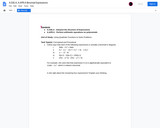Unrestricted Use
CC BY
Rating
0.0 stars

This is a Performance Task in which students are asked to determine equivalency in binomials.

Subject:
Mathematics
Material Type:
Assessment
Assessment Item
Interim/Summative Assessment
Author:
04/15/2019Restricted Use
Rating
0.0 stars

Digital algebra tiles that can be used on grids, dot grids, expression mats, equation mats, comparison mats, cornerpieces. Has x, y, x, y, xy, and unit tiles. This is not a lesson to use, but only the resource that can be used.

Subject:
Mathematics
Material Type:
Activity/Lab
Provider:
College Preparatory Mathematics
03/08/2017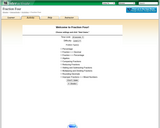Restricted Use
Rating
0.0 stars

This applet allows the students to learn to multiply, add, simplify and convert fractions, decimals and percents by playing a game.

Subject:
Mathematics
Material Type:
Activity/Lab
Diagram/Illustration
Formative Assessment
Interactive
Lesson Plan
Self Assessment
Simulation
Provider:
Shodor
02/14/2017Only Sharing Permitted
CC BY-NC-ND
Rating
0.0 stars

In this lesson students make connections between representations, clear parentheses, simplify, factorize, and use the arithmetic of polynomials in a purposive context.

Subject:
Mathematics
Material Type:
Formative Assessment
Lesson Plan
Self Assessment
Provider:
The Mathematics Assessment Resource Service
12/15/2016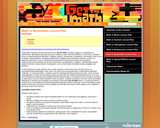Restricted Use
Rating
0.0 stars

Using video segments and web interactives from Get the Math, students engage in an exploration of mathematics, specifically reasoning and sense making, to solve real world problems. In this lesson, students focus on understanding the Big Ideas of Algebra: patterns, relationships, equivalence, and linearity; learn to use a variety of representations, including modeling with variables; build connections between numeric and algebraic expressions; and use what they have learned previously about number and operations, measurement, proportionality, and discrete mathematics as applications of algebra. Methodology includes guided instruction, student-partner investigations, and communication of problem-solving strategies and solutions. In the Introductory Activity, students view a video segment in which they learn how Elton Brand, an accomplished basketball player, uses math in his work and are presented with a mathematical basketball challenge. In Learning Activity 1, students solve the challenge that Elton posed in the video, which involves using algebraic concepts and reasoning to figure out the maximum height the basketball reaches on its way into the basket by using three key variables and Elton Brand's stats. As students solve the problem, they have an opportunity to use an online simulation to find a solution. Students summarize how they solved the problem, followed by a viewing of the strategies and solutions used by the Get the Math teams. In Learning Activity 2, students try to solve additional interactive basketball (projectile motion) challenges. In the Culminating Activity, students reflect upon and discuss their strategies and talk about the ways in which algebra can be applied in the world of sports and beyond.

Subject:
Career and Technical Education
Health Science
Mathematics
Material Type:
Assessment Item
Game
Interactive
Lesson Plan
Provider:
Next Generation Learning Challenges